Courses

# Forces on Fluid Elements Civil Engineering (CE) Notes | EduRev

## Civil Engineering (CE) : Forces on Fluid Elements Civil Engineering (CE) Notes | EduRev

The document Forces on Fluid Elements Civil Engineering (CE) Notes | EduRev is a part of the Civil Engineering (CE) Course Fluid Mechanics.
All you need of Civil Engineering (CE) at this link: Civil Engineering (CE)

Fluid Elements - Definition:

Fluid element can be defined as an infinitesimal region of the fluid continuum in isolation from its surroundings.

Two types of forces exist on fluid elements:

• Body Force: distributed over the entire mass or volume of the element. It is usually expressed per unit mass of the element or medium upon which the forces act.
Example: Gravitational Force, Electromagnetic force fields etc.
• Surface Force: Forces exerted on the fluid element by its surroundings through direct contact at the surface.

Surface force has two components:

• Normal Force: along the normal to the area
• Shear Force: along the plane of the area.

The ratios of these forces and the elemental area in the limit of the area tending to zero are called the normal and shear stresses respectively.
The shear force is zero for any fluid element at rest and hence the only surface force on a fluid element is the normal component.

Normal Stress in a Stationary Fluid
Consider a stationary fluid element of tetrahedral shape with three of its faces coinciding with the coordinate planes x, y and z.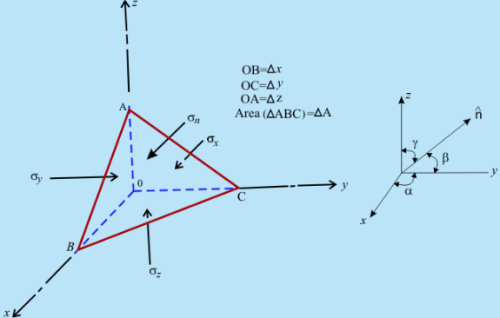Fig 3.1   State of Stress in a Fluid Element at Rest

Since a fluid element at rest can develop neither shear stress nor tensile stress, the normal stresses acting on different faces are compressive in nature.
Suppose, ΣFx, ΣFy and ΣFz  are the net forces acting on the fluid element in positive x,y and z directions respectively. The direction cosines of the normal to the inclined plane of an area ΔA are cos α, cos β and cos g.Considering gravity as the only source of external body force, acting in the -ve z direction, the equations of static equlibrium for the tetrahedronal fluid element can be written as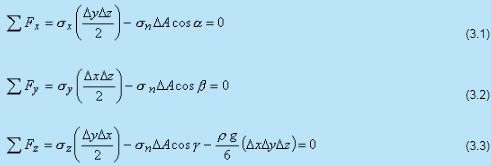where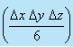= Volume of tetrahedral fluid element

Note: the pressure gradient is surface force that acts on the sides of the element. That must be balanced by gravity force, or weight of the element, in the fluid at rest. In addition to gravity, fluid in motion will have surface forces due to viscous stresses. Viscous forces, however, for fluid at rest are zero. The gravity force is body force, acting on the entire mass of the element:

dFgravity = ρgdx dy dz                fgravity = ρg

Pascal's Law of Hydrostatics
Pascal's Law

The normal stresses at any point in a fluid element at rest are directed towards the point from all directions and they are of the equal magnitude.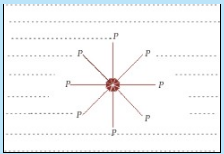Fig 3.2     State of normal stress at a point in a fluid body at rest

Derivation:

The inclined plane area is related to the fluid elements (refer to Fig 3.1) as follows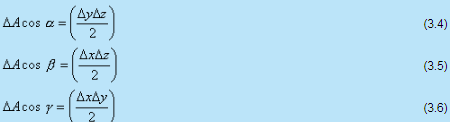Substituting above values in equation 3.1- 3.3 we get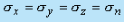(3.7)

Conclusion:
The state of normal stress at any point in a fluid element at rest is same and directed towards the point from all directions. These stresses are denoted by a scalar quantity p defined as the hydrostatic or thermodynamic pressure.
Using "+" sign for the tensile stress the above equation can be written in terms of pressure as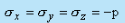(3.8)

Fundamental Equation of Fluid Statics
The fundamental equation of fluid statics describes the spatial variation of hydrostatic pressure p in the continuous mass of a fluid.

Derivation:
Consider a fluid element at rest of given mass with volume V and bounded by the surface S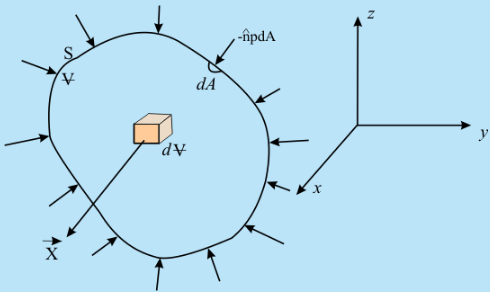Fig 3.3 External Forces on a Fluid Element at Rest

• The Resultant Body Force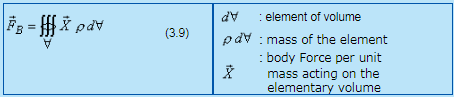•  The Resultant Surface Force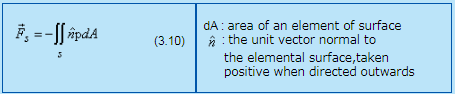Using Gauss divergence theorem, Eq (3.10) can be written as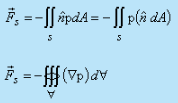(3.11)

For the fluid element to be in equilibrium , we have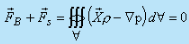(3.12)

The equation is valid for any volume of the fluid element, no matter how small, thus we get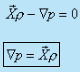(3.13)

This is the fundamental equation of fluid statics.

Fundamental Fluid Static Equations in Scalar Form
Considering gravity as the only external body force acting on the fluid element, Eq. (3.13) can be expressed in its scalar components with respect to a cartesian coordinate system (see Fig. 3.3) as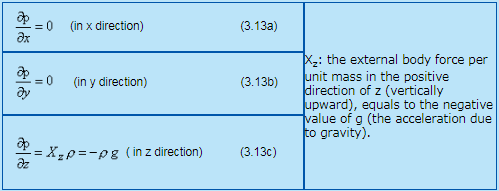From Eqs (3.13a)-(3.13c), it can be concluded that the pressure p is a function of z only.
Thus, Eq. (3.13c) can be re-written as,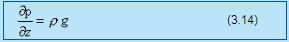Constant and Variable Density Solution
Constant Density Solution

The explicit functional relationship of hydrostatic pressure p with can be obtained by integrating the Eq. (3.14).
For an incompressible fluid, the density r is constant throughout. Hence the Eq. (3.14) can be integrated and expressed as
p = -p g z + c            (3.15)
where C is the integration constant.
If we consider an expanse of fluid with a free surface, where the pressure is defined as p = p0 ,which is equal to atmospheric pressure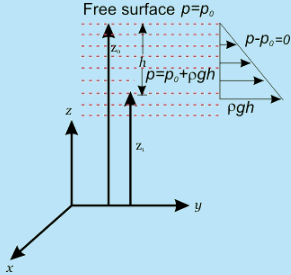Fig 3.4 Pressure Variation in an Incompressible Fluid at rest with a Free Surface

Eq. (3.15) can be written as, p- p=  p g (z- z1) = p g h    (3.16a)

Therefore, Eq. (3.16a) gives the expression of hydrostatic pressure p at a point whose vertical depression from the free surface is h.
Similarly, p- p= p g (z- z2) = pgh                                     (3.16b)

Thus, the difference in pressure between two points in an incompressible fluid at rest can be expressed in terms of the vertical distance between the points. This result is known as Torricelli's principle, which is the basis for differential pressure measuring` devices. The pressure p0 at free surface is the local atmospheric pressure.

Therefore, it can be stated from Eq. (3.16a), that the pressure at any point in an expanse of a fluid at rest, with a free surface exceeds that  of the local atmosphere by an amount rgh, where h is the vertical depth of the point from the free surface.

Variable Density Solution: As a more generalised case, for compressible fluids at rest, the pressure variation at rest depends on how the fluid density changes with height z and pressure p. For example this can be done for special cases of "isothermal and non-isothermal fluids".

Offer running on EduRev: Apply code STAYHOME200 to get INR 200 off on our premium plan EduRev Infinity!

## Fluid Mechanics

43 videos|70 docs|72 tests

,

,

,

,

,

,

,

,

,

,

,

,

,

,

,

,

,

,

,

,

,

;